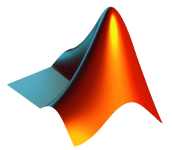### MATLAB introductory course

RTU scientific staff and faculty members coming across MATLAB for the first time are offered MATLAB introductory course. MATLAB is a high-level language and interactive environment for numerical computation, visualisation, and programming, which is widely used by scientists all over the world and increasingly at RTU. Since MATLAB offers many toolboxes, efficient use and application thereof in scientific work requires knowledge of the MATLAB language and skills in using the MATLAB command prompt window. This course is provided by lecturer Dr. sc. ing. Māris Tērauds for participants in groups of 10–15.### Content

The course covers basic language constructions, functions, scripts, data filtering and cross-section design, as well as shows relational operations, symbolic math, data import and export and how graphs can be adapted to your scientific results.

As MATLAB is becoming more and more user-friendly, a part of operations can be carried out using computer mouse only. Nevertheless, participants of this course will work in command prompt window and not with a mouse.

### Agenda

• Introduction to MATLAB, basic commands, basic objects
• Processing measurement data in MATLAB, data interpolation, approximation, regression and import
• Graphic objects in MATLAB, handle graphics
• Working with symbolic math
• Functions, scripts, data filtering, cross-section design
• Cycles in MATLAB, parallel computing in MATLAB
• Other MATLAB objects, structures, cell matrixes, automatic preparation of tasks for students, creating of reports
• Creation of MATLAB interface (buttons, etc.), guide, uicontrol